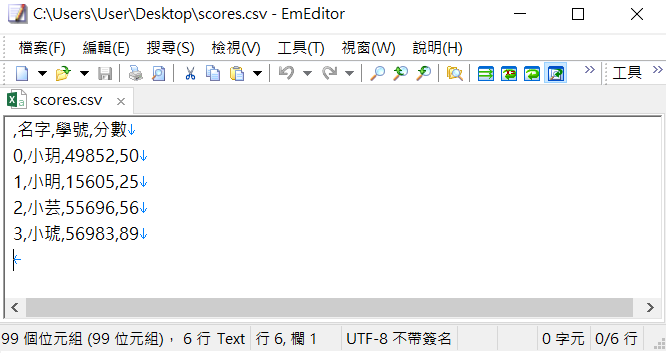#0

## 【python好用模組-pandas】好用的資料處理工具

Pandas就是把Excel的表格觀念丟到Python，

Spyder->python3.7
pandas版本0.23.4

# 安裝

pandas不是python的內建模組，

# 引用模組

``````import pandas as pd
``````

# 創建資料

1. Series 欄位(一維度)
2. DataFrame 表格（二維度）
(三維以上的表格似乎不常用，先略過)

## Series 欄位

index預設是0，1，2，3，…

``````import pandas as pd
L = list(range(1,20,3))
s1 = pd.Series(L)
print(s1)
``````

``````0     1
1     4
2     7
3    10
4    13
5    16
6    19
dtype: int64
``````

`pd.Series()`多加一個index參數放list即可

``````import pandas as pd
L = list(range(1,20,3))
s1 = pd.Series(L, index = [chr(ord('a')+i) for i in range(7)])
print(s1)
``````

``````a     1
b     4
c     7
d    10
e    13
f    16
g    19
dtype: int64
``````

``````import pandas as pd
s1 = pd.Series({'小狗': 50, '小貓': 60, '小熊': 25})
print(s1)
``````

``````小狗    50

dtype: int64
``````

## DataFrame 表格

``````import pandas as pd
scores = [{"姓名":"小華", "數學":90, "國文":80},
{"姓名":"小明", "數學":70, "國文":55},
{"姓名":"小李", "數學":45, "國文":75}]
score_df = pd.DataFrame(scores)
print(score_df)

scores = {"姓名":["小華","小明","小李"],
"國文":[80,55,75],
"數學":[90,70,45]}
score_df = pd.DataFrame.from_dict(scores)
print(score_df)
``````

``````   國文  姓名  數學
0  80  小華  90
1  55  小明  70
2  75  小李  45
姓名  國文  數學
0  小華  80  90
1  小明  55  70
2  小李  75  45
``````

# 讀取csv資料

``````import pandas as pd
scores = pd.read_csv('scores.csv', index_col = 0)
print(scores)
``````

``````   名字     學號  分數
0  小玥  49852  50
1  小明  15605  25
2  小芸  55696  56
3  小琥  56983  89
``````# 截取部分資料- loc, iloc, at, iat

pandas的取值有四種方法- loc, iloc, at, iat，
i是index的意思。沒有i的則是直接以index名稱取值

``````   名字     學號  分數
0  小玥  49852  50
1  小明  15605  25
2  小芸  55696  56
3  小琥  56983  89
``````

``````import pandas as pd
scores = pd.read_csv('scores.csv', index_col = 0)
print(scores.iloc[[0,2], 0:2])
print(scores.loc[[0,2], ['名字', '學號']])
``````

``````   名字     學號
0  小玥  49852
2  小芸  55696
``````

``````import pandas as pd
scores = pd.read_csv('scores.csv', index_col = 0)
print(scores.at[1, '名字'])
print(scores.iat[1, 0])
``````

``````小明
``````

# 截取部分資料- 中括號

``````import pandas as pd
scores = pd.read_csv('scores.csv', index_col = 0)
print(scores['名字'])
print(scores[['名字']])
print(scores[['名字', '學號']])
``````

``````0    小玥
1    小明
2    小芸
3    小琥
Name: 名字, dtype: object

名字
0  小玥
1  小明
2  小芸
3  小琥

名字     學號
0  小玥  49852
1  小明  15605
2  小芸  55696
3  小琥  56983
``````

``````import pandas as pd
scores = pd.read_csv('scores.csv', index_col = 0)
print(scores[0:2]) #取得第0~2(不包含2)個學生的資料
``````

``````   名字     學號  分數
0  小玥  49852  50
1  小明  15605  25
``````

## 條件篩選(與numpy相似)

pandas亦可以指定將滿足條件的資料篩選出來，

``````import pandas as pd
scores = pd.read_csv('scores.csv', index_col = 0)
print(scores[scores['分數']>50])
``````

``````   名字     學號  分數
2  小芸  55696  56
3  小琥  56983  89
``````

# 資料行、列互換

column則會記錄「屬性」，

``````import pandas as pd
scores = pd.read_csv('scores.csv', index_col = 0)
print(scores.T)
``````

``````        0      1      2      3

``````

# 輸出excel檔

`.to_excel('檔案名稱.xls')`就好了，

``````import pandas as pd
scores = pd.read_csv('scores.csv', index_col = 0)
scores.to_excel('table.xls')
``````